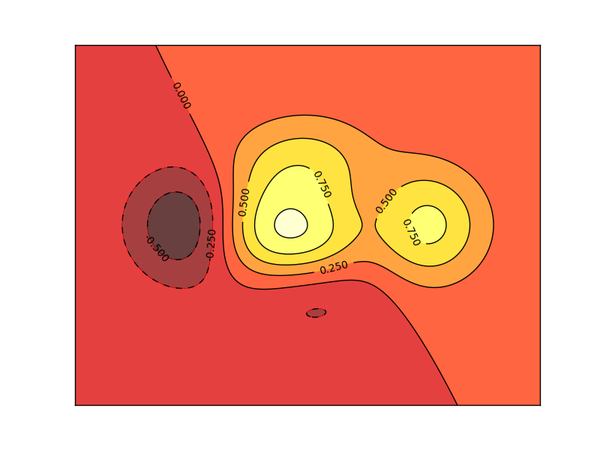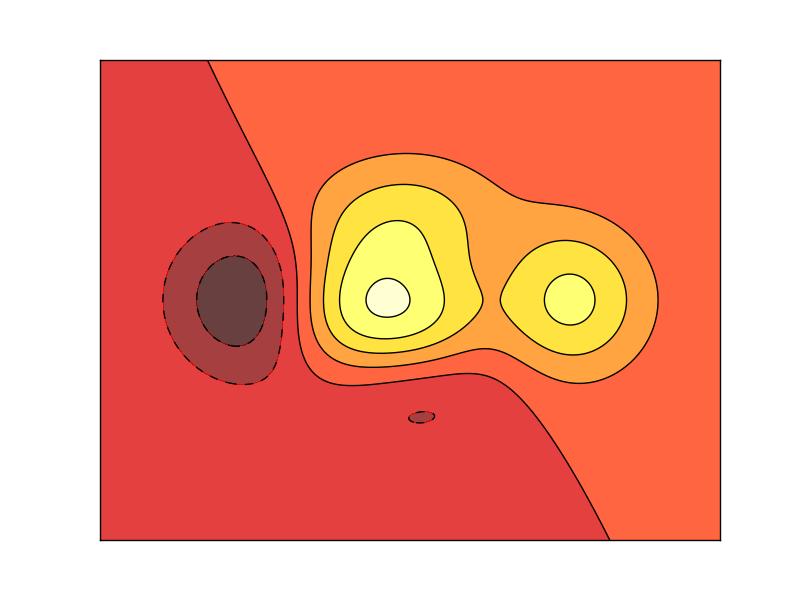# Matplotlib contours 等高线图

728℃## 画等高线

``````import matplotlib.pyplot as plt
import numpy as np

def f(x,y):
# the height function
return (1 - x / 2 + x**5 + y**3) * np.exp(-x**2 -y**2)

n = 256
x = np.linspace(-3, 3, n)
y = np.linspace(-3, 3, n)
X,Y = np.meshgrid(x, y)``````

``````# use plt.contourf to filling contours
# X, Y and value for (X,Y) point
plt.contourf(X, Y, f(X, Y), 8, alpha=.75, cmap=plt.cm.hot)``````

``````# use plt.contour to add contour lines
C = plt.contour(X, Y, f(X, Y), 8, colors='black', linewidth=.5)``````## 添加高度数字

``````plt.clabel(C, inline=True, fontsize=10)
plt.xticks(())
plt.yticks(())``````